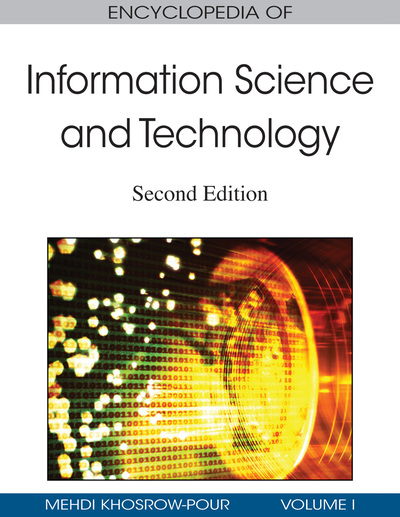# Quantum Cryptography Protocols for Information Security

Göran Pulkkis (Arcada Polytechnic, Finland) and Kaj J. Grahn (Arcada Polytechnic, Finland)
DOI: 10.4018/978-1-60566-026-4.ch510

## Abstract

Quantum cryptography will have a severe impact on information security technology. The objective of this article is to present state-of-the-art and future possibilities of two quantum cryptography protocol types. These protocols are for absolutely secure distribution of symmetric encryption/ decryption keys and for creating secure digital signatures.
Chapter Preview
Top

## Information Representation With Quantum States

Quantum states are energy levels of molecules, atoms, and photons. Two quantum states for which a state transition exists can be used to represent an information bit, if the energy levels of both states can be measured. A bit defined by quantum states is called a quantum bit or qubit. However, quantum states are probabilistic. When the energy level of a molecule, an atom, or a photon is measured, the outcome is one of all possible energy levels, and each possible outcome is associated with a probability. The sum of the probabilities of all possible measurement outcomes is, of course, 1. A qubit is thus also probabilistic. The binary values 0 and 1 are represented by two possible quantum states of a qubit. If the measurement probabilities of these two quantum states are p0 and p1, then p0+p1=1.

## Key Terms in this Chapter

Raw Key: Two parties agree on a shared binary secret from a sequence of randomly polarized photons.

Avalanche Photodiode: A photodetector which can be regarded as the semiconductor analog to a photomultiplier.

QPN: A registered abbreviation for Quantum Private Network, which is a VPN link where session key agreement is based on a QKD protocol.

Polarization: The plane in which an electromagnetic wave propagates.

Photon: A discrete packet of electromagnetic energy.

Teleportation: Transfer of an unknown qubit state.

Quantum Gate: A device for changing the state of one or of multiple qubits.

## Complete Chapter List

Search this Book:
Reset